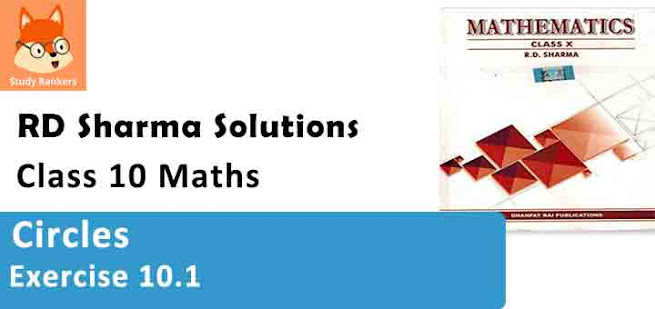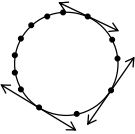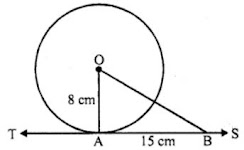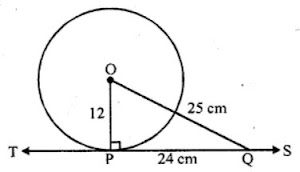# RD Sharma Solutions Chapter 10 Circles Exercise 10.1 Class 10 MathsChapter Name RD Sharma Chapter 10 Circles Book Name RD Sharma Mathematics for Class 10 Other Exercises Exercise 10.1Exercise 10.2MCQ and VSAQs Related Study NCERT Solutions for Class 10 Maths

### Exercise 10.1 Solutions

1. Fill in the blanks :
(i) The common point of a tangent and the circle is called ……
(ii) A circle may have …… parallel tangents.
(iii) A tangent to a circle intersects it in ……. point(s).
(iv) A line intersecting a circle in two points is called a ……
(v) The angle between tangent at a point on a circle and the radius through the point is ……

Solution

(i) The common point of a tangent and the circle is called the point of contact.
(ii) A circle may have two parallel tangents.
(iii) A tangents to a circle intersects it in one point.
(iv) A line intersecting a circle in two points is called a secant.
(v) The angle between tangent at a point, on a circle and the radius through the point is 90°.

2. How many tangents can a circle have ?

SolutionA circle can have infinitely many tangents as there can many point lie on the circumference of the circle.

3. O is the centre of a circle of radius 8 cm. The tangent at a point A on the circle cuts a line through O at B such that AB = 15 cm. Find OB.

Solution

Radius OA = 8 cm, ST is the tangent to the circle at A and AB = 15 cmOA ⊥ tangent TS
In right ∆OAB,
OB² = OA² + AB² (Pythagoras Theorem)
⇒ OB² = (8)² + (15)²
⇒ OB² = 64 + 225
⇒ OB² = 289
⇒ OB² = (17)²
⇒ OB = 17 cm

4. If the tangent at a point P to a circle with centre O cuts a line through O at Q such that PQ = 24 cm and OQ = 25 cm. Find the radius of the circle.

Solution

OP is the radius and TS is the tangent to the circle at POQ is a line
OP ⊥ tangent TS
In right ∆OPQ,
OQ² = OP² + PQ² (Pythagoras Theorem)
⇒ (25)² = OP² + (24)²
⇒ 625 = OP² + 576
⇒ OP² = 625 – 576 = 49
⇒ OP² = (7)²
⇒ OP = 7 cm
Hence, radius of the circle is 7 cm.